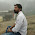# C++ program for Round Robin Scheduling Algorithm.

Problem:- Implementing Round Robin Scheduling Algorithm in C++ or Round Robin Scheduling Algorithm in C++ or Program for Round Robin scheduling or Round Robin Scheduling Program in C or round robin scheduling algorithm or Round Robin algorithm c++ code or Write Program In C++ For Round Robin Scheduling or Review of Round robin code in c++ or C program for Round Robin CPU Scheduling Algorithm.

Check This:- Hacker rank solution for Strings, Classes, STL, Inheritance in C++.

Explanation:- We can Understand Round Robin Scheduling Algorithm by taking an example Suppose there is 4 process. and each process comes at the same time so based on FIFO (First in First Out) scheduler keep all process in Ready Queue and for given time Slice each process will be executed until all process finish. Let's take an example and try to understand How Round Robin Works.

Example:-

Quantum = 4

Process    Burst Time     Arrival Time

P1              5                     0
P2              3                     0
P3              8                     0
P4              9                     0

Now Schedular keeps all process in Ready Queue and based on FIFO (First in First Out) send the first process for execution.

P1   P2   P3   P4   P1   P3 P4 P4
0 4 7    11 12    16 20    21

Processes    Burst time      Waiting time        Turn around time
1 5 11                          16
2 3 4                            7
3 8 12                          20
4 9 16  25

Average waiting time = 10.75
Average turn around time = 17

1. What is Round Robin Scheduling Algorithm?.
2. How to Calculate Turn Around Time?.
3. How to Calculate Waiting Time?.
4. Example.

What is Round Robin Scheduling Algorithm?.

Round Robin is a primitive Scheduling Algorithm and most important and commonly used scheduling algorithm for CPU. In Round Robin Scheduling Algorithm each process has its own execution time that is called " Quantum " and after Quantum time next process start executes for given Quantum time and so on once a cycle complete again process execution start from first, process and repeat the process again and again and for saving a current state of process Context switching is used.

1. Round Robin is a primitive Scheduling Algorithm.

2. Round Robin follow FIFO (First in First Out) Principle.

3. For executing each process in Round Robin Time cluster or time Slice provides, so a process can execute for a particular given amount of time, the given time is called Quantum.

4. After Quantum time for saving a state of each process Context switching is used.

5. Round Robin is Great use for fully Utilization of a CPU and Multitasking.

6. Scheduler always needs to keep ready next process ready in ready Queue or Queue for execution in CPU so we can say that scheduler play an important role in round robin.

7. After Quantum Time for each process same step repeat again and again.

How to Calculate Turn Around Time?.

Turn Around Time = Completion Time – Arrival Time

With the help of this formula, we can calculate a Turn Around Time of all process in Queue.

How to Calculate Waiting Time?.

Waiting Time = Turn Around Time – Burst Time

This formula is used for calculating the waiting time for rest of process.

Also Check:- Geeksforgeeks solution for School, Basic, Easy, Medium, Hard in C++.

Extreme Recommended:- Like our Facebook Page or Join our Facebook Group and Google plus Community for up-to-date for a new post or if you have any Query you can ask there with lots of coders also suggest to your Friends to join and like our page, so we can help our community, and don't forget to Subscribe. Enter your Email and click to subscribe.

Note:- Universal Code will be updated Soon.

Solution:-

#include<iostream>
#include<cstdlib>
#include<queue>
#include<cstdio>
using namespace std;

typedef struct process
{
int id,at,bt,st,ft,pr;
float wt,tat;
}process;

process p,p1,temp;
queue<int> q1;

int accept(int ch);
void turnwait(int n);
void display(int n);
void ganttrr(int n);

int main()
{
int i,n,ts,ch,j,x;
p.tat=0;
p.wt=0;
n=accept(ch);
ganttrr(n);
turnwait(n);
display(n);
return 0;
}

int accept(int ch)
{
int i,n;
printf("\nEnter the no. of process: ");
scanf("%d",&n);
if(n==0)
{
printf("\nInvalid no. of process");
exit(1);
}
for(i=1;i<=n;i++)
{
printf("\nEnter the arrival time for process P%d: ",i);
scanf("%d",&p[i].at);
p[i].id=i;
}
for(i=1;i<=n;i++)
{
printf("\nEnter the burst time for process P%d: ",i);
scanf("%d",&p[i].bt);
}
for(i=1;i<=n;i++)
p1[i]=p[i];
return n;
}

void ganttrr(int n)
int i,ts,m,nextval,nextarr;
nextval=p1.at;
i=1;
cout<<"\nEnter time slice: ";
cin>>ts;
for(i=1;i<=n && p1[i].at<=nextval;i++)
q1.push(p1[i].id);
while(!q1.empty())
{
m=q1.front();
q1.pop();
if(p1[m].bt>=ts)
nextval=nextval+ts;
else
nextval=nextval+p1[m].bt;
if(p1[m].bt>=ts)
p1[m].bt=p1[m].bt-ts;
else
p1[m].bt=0;
while(i<=n&&p1[i].at<=nextval)
{
q1.push(p1[i].id);
i++;
}
if(p1[m].bt>0)
q1.push(m);
if(p1[m].bt<=0)
p[m].ft=nextval;
}
}

void turnwait(int n)
{
int i;
for(i=1;i<=n;i++)
{
p[i].tat=p[i].ft-p[i].at;
p[i].wt=p[i].tat-p[i].bt;
p.tat=p.tat+p[i].tat;
p.wt=p.wt+p[i].wt;
}
p.tat=p.tat/n;
p.wt=p.wt/n;
}

void display(int n)
{
int i;
cout<<"\n\n-------------------TABLE----------------------------------\n";
printf("\nProcess\tAT\tBT\tFT\tTAT\t\tWT");
for(i=1;i<=n;i++)
printf("\nP%d\t%d\t%d\t%d\t%f\t%f",p[i].id,p[i].at,p[i].bt,p[i].ft,p[i].tat,p[i].wt);
cout<<"\n\n-----------------------------------------------------------";
printf("\nAverage Turn Around Time: %f",p.tat);
printf("\nAverage Waiting Time: %f\n",p.wt);
}

Output:-

1.1.